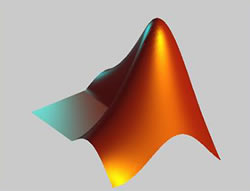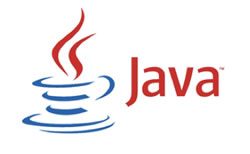# Difference between MATLAB and Java

##### Key Difference: MATLAB is a computer language for supporting interactive numerical mathematics developed in the 1970s. On the other hand, Java is a programming language and computing platform which was first released by Sun Microsystems in 1995.

MATLAB and Java, both are computing languages that are usually found in many corporate structures. It’s a very detailed ongoing series but lets try to discuss the basic points which will be helpful to understand the topic.MATLAB is a computer language for supporting interactive numerical mathematics, which was developed in the late 1970s by Cleve Moler, the chairman of the computer science department at the University of New Mexico. The "Mat" in MATLAB stands for matrix, as MATLAB has strong support for matrix computations. It also has good support for plotting graphs, and it's for that purpose that it is used with the Lunar Lander project. MATLAB is mostly a company based program. It can be included in home PCs but the user will require a basic knowledge for it.

The problem with MATLAB is that it is terribly expensive. If a student or a middle man needs to include MATLAB in their computer, it will be very costly for them.

Here were five primary goals in the creation of the Java language:

• It should be "simple, object-oriented and familiar"
• It should be "robust and secure"
• It should be "architecture-neutral and portable"
• It should execute with "high performance"
• It should be "interpreted, threaded, and dynamic"Java is a programming language and a computing platform which was first released by Sun Microsystems in 1995. Java is the most preferable program that people include, because it is very familiar, object-oriented and modern. C is included in Java, which is the lower level programming language. There are lots of applications and websites that will not work unless you have installed Java, and more are created every day. Java is fast, secure, and reliable.

Here are some of the differences between MATLAB and Java:

• MATLAB has much more support for high-level mathematical operations, like matrix multiplication. You could write (or find) libraries to do these operations in Java, but it's a lot more work.
• MATLAB is interpreted (like Dr. Java), not compiled like Java.  This makes it easy to experiment interactively.
• MATLAB runs more slowly than Java, except for doing built-in matrix operations like finding values (for which MATLAB is usually faster).